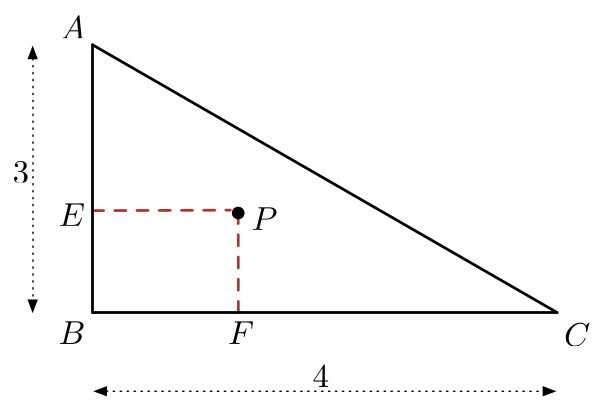# A Peculiar Locus Area

Calculus Level 2$\triangle ABC$ is constructed such that $AB=3$, $BC=4$, and $\angle ABC=90^{\circ}$. Point $P$ is chosen inside $\triangle ABC$, and points $E$ and $F$ are drawn such that they form line segments with $P$ that are perpendicular to sides $AB$ and $BC$, respectively. If $\mathbf{L}$ is the locus of all points $P$ such that $[PEBF]\ge 1$, then find the value of $\left\lfloor 1000\dfrac{[\mathbf{L}]}{[ABC]}\right\rfloor$

$\text{Details and Assumptions:}$

$[\mathbf{N}]$ means the area of the locus $\mathbf{N}$, and $[PQRS]$ means the area of $PQRS$. $\lfloor x \rfloor$ is the floor function.

×